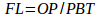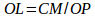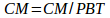# Calculation of Financial LeverageThe financial leverage (FL) is the relationship between the company’s earnings before interest and taxes or operating profit (OP) and the profit available to shareholders before tax (PBT).Assignment:

Arvind Ltd. is having the following informations. Calculate financial leverage
opening leverage and combined leverage.
Sales 50,000 units Rs. 10 each
UC Rs. 6 Per Unit
FC Rs. 1,00,000
Interest 8 of 5,00,000

The operating profit (OP) will be calculated using statement of profit as shown below.

 Total Per Unit Units Sold 50,000 Sales revenue (units sold x price per unit), R s. 5,00,000 10 Less: Variable cost (UC), R s. 3,00,000 6 Less: Fixed cost (FC), R s. 1,00,000 Operating profit (OP), R s. 1,00,000 Less: Interest 8% of 5,00,000 debt, R s. 40,000 Profit available for shareholders (PBT), R s. 60,000

TotalPer Unit
Financial leverage equals 1.67
FL = 1,00,000/60,000 = 1.67

Calculation of Operating Leverage

The operating leverage (OL) is the ability to use fixed expenses and can be computed contribution margin (CM) divided by operating profit (OP):The contribution margin (CM) will be calculated using statement of profit as shown below

 Total Per Unit Units Sold 50,000 Sales revenue (units sold x price per unit), R s. 5,00,000 10 Less: Variable cost (UC), R s. 3,00,000 6 Contribution margin (CM), R s. 2,00,000 4

OL = 2,00,000/1,00,000 = 2.00

Operational leverage equals 2.00

Calculation of Combined Leverage

The combined leverage (CM) shows the relationship between the company’s sales (CM) and profit before tax (PBT). The combined leverage is calculated using the two formulas:CM = 1.67 x 2.00 =3.33 or CM = 2,00,000/60,000 =3.33

Combined leverage equals 3.33